### Home > CC3 > Chapter 6 > Lesson 6.2.2 > Problem6-63

6-63.
1.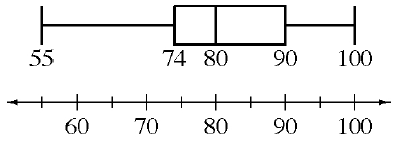The box plot at right shows the different grades (in percents) that students in Ms. Sanchez’s class earned on a recent test. Homework Help ✎

1. What was the median score on the test? What were the highest and lowest scores?

2. Did most students earn a particular score? How do you know?

3. If Ms. Sanchez has 32 students in her class, about how many students earned a grade of 80% or higher? About how many earned more than 90%? Explain how you know.

4. Can you tell if the scores between 80% and 90% were closer to 80% or closer to 90%? Explain.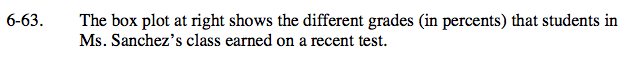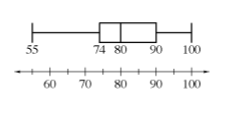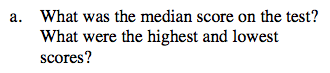Do you remember how to read a box plot?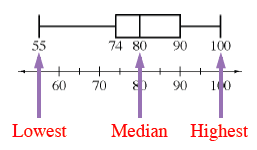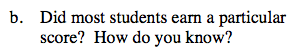Can this be determined from a box plot?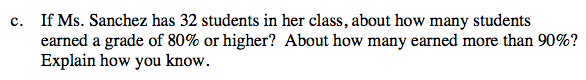Since 80% is the median, half of the class earned 80% or higher and half earned lower than 80%.

$\frac{1}{4}\text{ of the class earned 90\%}$

16 students earned 80% or higher, 8 students earned 90% or higher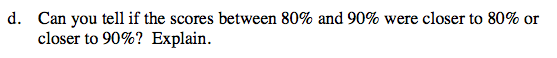Can this be determined from a box plot?